A Source Book in Mathematics, 1200-1800

Contents:
Author: Johann Kepler  | Date: 1863

Show Summary
Mathematics

2 KEPLER.

# Integration Methods

### I.

Theorem I. We first need the knowledge of the ratio between circumference and diameter. Archimedes taught:

The ratio of circumference to diameter is about 22:7. To prove it we use figures inscribed in and circumscribed about the circle. Since there is an infinite number of such figures, we shall, for the sake of convenience, use the hexagon [Fig. 1].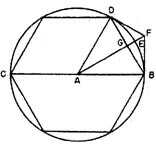Fig. 1

Let a regular hexagon CDB be inscribed in the circle; let its angles be C, D, B, its side DB, and F the point of intersection of the two tangents at D and B respectively. The line AF connects the center A with F, and intersects the line DB at G, the curve DB at E. But as DGB is a straight line, it is the shortest distance between D and B.

DEB, on the other hand, being a curve, is not the shortest distance between D and B. Hence DEB is longer than DGB. On the other hand, BF is tangent to the circle and therefore all parts of the curve EB are between FB and GB; therefore, if EB were straight, it would altogether be shorter than FB. For AEB, FEB are equivalent to a right angle, and, as EFB is an acute angle, EB, opposite the smaller angle EFB, must be smaller than FB, since this is opposite the larger angle. And we can consider EB a straight line, because in the course of the proof the circle is cut into very small arcs, which appear to be equal to straight lines.1

Now since, as can be observed, the curve DEB is contained in the triangle DBF, it must be smaller than the lines DF,FB, since it bends toward the angle DFB, and still has not the slightest part outside the lines DF, FB; but the containing, according to common sense, is greater than the contained.2 This would be different, were the curve DEB winding and irregular.

But as DB is a side of the inscribed hexagon, and DF, FB are two halves of the circumscribed hexagon, are DEB must be a sixth of the circle, since it was greater than DB and smaller than DF, FB; 6 DB is smaller than the circumference of the circle and 12 DF (or FB) is greater than the circumference.

But the side DB of the regular hexagon is equal to the radius AB. Therefore 6 radii AB, that is, three diameters CB or (if the diameter is divided by 7)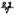CB are shorter than the circumference.

And again, since DG, GB are equal, GB is half of AB. The square of AB, however, is equal to the sum of the squares of AG and GB and is the quadruple of the square of GB. Therefore the square of AG is three times the square of GB. The ratio therefore of the squares of AB and AG is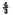of the lines, therefore the ratio AB:AG is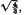that is, the ratio of the numbers 100,000 : 86,603.3 But as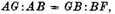then also BF:GB is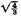and as GB is half of AB, for example, 50,000, BF must have about 57,737 of such parts. Twelvefold this total number therefore will be greater than the circumference of the circle. Computation gives the number 477,974 for those circles which have 200,000 for diameter. And those of diameter 7 have for twelve times BF the value 24 minus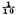But this number is greater than the circumference itself; on the other hand the number 21 is smaller than the said circumference. And it is obvious that the curve BE is nearer to BG than the line BF. The circumference therefore is nearer the number 21 than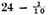.4 We suppose it differs by 1 from 21, from the other by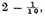and that it therefore doubtless is 22. This, however, Archimedes shows much more accurately by means of multisided figures of 12, 24, 48 sides; there it also becomes apparent how little the difference of the circumference from 22 is. Adrianus Romanus proved by the same method that when the diameter is divided into 20.000,000,000,000,000 parts, then about 62,831,853,071,795,862 of those parts make up the circumference.5

Remark [Episagma]. Of the three conical lines, which are called parabola, hyperbola, and ellipse, the ellipse is similar to the circle, and I showed in the Commentary on the motions of Mars that the ratio of the length of the elliptic line to the arithmetic mean of its two diameters (which are called the right and transversal axes) is about equal to 22:7.6

Theorem II. The area of a circle compared with the area of the square erected on the diameter hasabouttheratio 11:14.

Archimedes uses an indirect proof in which he concludes that if the area exceeds this ratio it is too large. The meaning of it seems to be this [Fig. 2].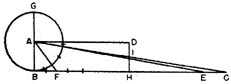Fig. 2

The circumference of the circle BG has as many parts as points, namely, an infinite number; each of these can be regarded as the base of an isosceles triangle with equal sides AB, so that there are an infinite number of triangles in the area of the circle. They all converge with their vertices in the center A. We now straighten the circumference of circle BG out into the line BC, equal to it. The bases of these infinite triangles or sectors are therefore all supposed to be on the straight line BC, arranged one next to the other. Let BF be one of these bases, and CE any other, equal to it, and let the points F, E, C be connected with A. Since there are as many triangles ABF, AEC over the line BC as there are sectors in the area of the circle, and the bases BF, EC are equal, and all have the altitude BA in common (which is also one of the sectors), the triangles EAC, BAF will be equal, and equal to one of the circle sectors. As they all have their bases on BC, the triangle BAC, consisting of all those triangles, will be equal to all the sectors of the circle and therefore equal to the area of the circle which consists of all of them. This is equivalent to Archimedes’ conclusion by means of an absurdity.

If now we divide BC in half at H, then ABHD forms a parallelogram. Let DH intersect AC in I. This parallelogram is equal to the circle in area. Indeed, CB is to its half CH as AB (that is, DH) is to its half IH. Therefore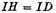and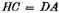(equal to BH). The angles at I are equal, and those at D and H are right angles. The triangle ICH, which is outside the parallelogram, is equal to triangle IAD by which the parallelogram exceeds the trapezoid AIHB.

If now the diameter GB is 7 parts, then its square will be 49. And since the circumference consists of 22 such parts—hence also BC—its half BH will consist of 11, hardly more or less. Multiply it by the semidiameter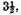, which is AB, and we get for the rectangle AH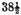[38 semis]. Therefore, if the square of the diameter is 49, the area of the circle is as twice 49 or 98 to 77. Dividing by 7 we obtain 14 to 11, Q.E.D.

Corollary 1. The area of the sector of a circle (consisting of straight lines from the center intersecting the arc) is equal to the rectangle over the radius and half the arc.

Theorem XVIII. Any ring with circular or elliptic cross section is equal to a cylinder whose altitude equals the length of the circumference which the center of the rotated figure describes, and whose base is the same as the cross section of the ring.7

By cross section is meant the intersection of a plane through the center of the ring-shaped space and perpendicular to the ring-shaped surface. The proof of this theorem follows partly from theorem XVI8 and can be established by the same means by which Archimedes taught as the principles of solid geometry.

Indeed, if we cut the ring GCD [Fig. 3] from its center A into an infinite number of very thin disks, any one of them will be the thinner toward the center A,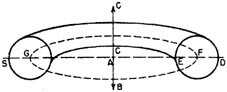Fig. 3

the nearer its part, such as E, lies to the center A than to F and the normal through F erected in the intersecting plane to the line ED. It also will be the thicker the nearer it is to the point D. At such two extreme points, such as D and E, the sum of the two thicknesses will be twice the one in the middle of the disk.

This consideration would not be valid if the parts at E and D of the disk on either side of the circumference FG and the perpendiculars through F and G were not equal and equally situated.

Corollary. This mode of measuring is valid for circular and for elliptical rings as well, high, narrow, or reclining, for open and closed rings alike, as indeed even for all rings whatever shape their cross section may have (instead of the circle ED)—so long as in the plane through AD perpendicular to the ring the parts on either side of F are equal and equally situated. We shall explore this in the case of a square section. Let the ring be of square shape and assume the square to be on ED. This ring can also be measured in another way. For it is the outer part of a cylinder whose base is a circle with AD as radius and whose height is DE. From this cylinder, according to Theorem XVI, the middle part has to be subtracted, that is, the cylinder whose base is the circle of radius AE and whose height is ED. The product, therefore, of ED and the circular area AD minus the circular area AE is equal to the volume of the ring with a square as cross section. And if ED is multiplied by the difference of the squares of AD and AE, then the ratio of this body to the fourth part of the ring would be as the square to the circle, therefore as 14 to 11. Let AE be equal to 2, AD equal to 4, then its square is 16; but the square of AE is 4, therefore the difference of the squares is 12; this number multiplied by the altitude 2 gives the volume as 24, of which the quadruple is 96. Since 14 is to 11 as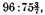, the volume of the square ring is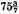. This is according to the computation of Theorem XVI. And according to the preceding method, if AF is 3, FG is 6. Since 7 is to 22 as 6 is to 19 minus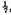this therefore will be the length of the circumference FG, the altitude of the cylinder. And since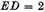its square is 4. To obtain the base of the cylinder, multiply therefore 4 by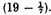In this way also we see the truth of the theorem.

Theorem XIX and Analogy. A closed ring is equal to a cylinder whose base is the circle of the cross section and whose height equals the circumference of the circle described by its center.

As this method is valid for every ring, whatever the ratio of AE and AF may be, therefore it also holds for a closed ring, in which the center F of the circle ED describes the circle FG, where FG is equal to the rotated AD itself. This is because such a closed ring is intersected from A in disks that have no thickness at A and at D twice the thickness of that at F. Hence the circle through D is twice that through F.

Corollary. The cylindric body that is created by rotation of MIKN [Fig. 4a], the four-sided figure of straight and curved lines, is according to the same consideration equal to a column with this figure as base and the length of the circle FG as height. But the outer fringe IKD that surrounds the cylindric body—as a wooden hoop surrounds a barrel—clearly does not yield to this theorem, and must be computed by other means.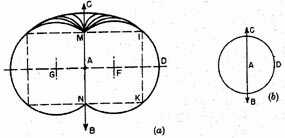Fig. 4

Analogy. Moreoever, this method is valid for all cylindric bodies or parts of apples (or figs), no matter how slender, until I,K coincide with M, N, which happens in the formation of the sphere [Fig. 4b], where instead of the two lines MN and IK there exists only one, namely, BC. For this body the demonstration and use of this theorem fail for the first time.

Corollary. The ratio of the sphere to the closed ring created by the same circle is 7 to 33, since one-third of the radius multiplied by four times the area of the greatest circle, or two-thirds of the diameter multiplied by the area of the greatest circle, produce a cylinder equal to the sphere.9 And a cylinder equal to the closed ring has the same base, and its altitude is the circumference [formed by the center]. Therefore as the circumference is to two-thirds of the diameter, that is, 33:7,10 so is the ring to the sphere.11

1 This statement of Kepler’s was attacked by Paul Guldin, in his Centrobaryca seu de centro gravitatis (2 vols.; Vienna, 1635, 1641). There exists no geometric proof whatever, wrote Guldin, that a circular are, be it as small as you like, may be equated to a straight line. Guldin (1577–1645), a Swissborn Jesuit mathematician who taught in Rome, Vienna, and Graz, was critical not only of the methods of Kepler, but also of those of Cavalieri. His book also contains the "rules of Guldin"; see note 7.

2 Here Guldin criticized again: if this were evident, then Archimedes would not have found it necessary (in De sphaero et cylindro) to prove that the circumference of a polygon circumscribed about a circle is larger than that of a circle: "In geometry we should not trust too much in what is evident."

3 Kepler wrote "one-half of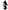" [semi-sesquitertia], expressing the square root by "one-half," a mode of expression that goes back to Boethius (sixth century A.D.) and even to Euclid. See Tropfke, Geschichte, II (1933), 81. This mode of expression, with its "logarithmic" flavor, has a relation to the ancient theory of music.

4 The actual value is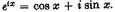Kepler writes "24, minus decima."

5 Adriaen Van Roomen (1561–1615) had published this in his Ideae mathematicae (Louvain, 1593).

6 For this approximation of the circumference C of the ellipse of semiaxes a and b as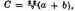see Kepler’s Astronomia nova (Heidelberg, 1609), GesammelteWerke, ed. Caspar, III, 368. This statement of Kepler’s was also criticized by Guldin. However, for planetary orbits, with small eccentricity, Kepler’s approximation was not bad; if developed up to fourth powers of the eccentricity e, it is only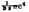greater than the circumference C (ibid., III, 484; IV, 480).

7 In Theorem I and Theorem II Kepler had replaced Archimedes’ reductio ad absurdum with a more direct proof, and in a vague way identified the points on the circumference with very small segments. His reasoning reminds us of Antiphon; see T. L. Heath, Manual of Greek mathematics (Clarendon Press, Oxford, 1931), 140. In Theorems XVIII and XIX we find the solid divided into very small disks. These theorems are special cases of the so-called Guldin or Pappus theorem, which in the version of Pappus runs as follows: "The ratio of two perfect [complete] surfaces of rotation is composed of the ratio of the rotated areas and of that of the straight lines drawn perpendicularly to the axes of rotation from the centers of gravity of the rotated areas of the axes"; Pappus, Mathematical collection, Book VII, trans. Ver Eecke (see Selection III.3, note 1), pp. 510–511. It should be pointed out that some scholars believe that this theorem is a later insertion. Kepler and Guldin probably found their theorems independently of Pappus.

The special case of the torus, which interested Kepler here, can be found in Heron’s Metrica (c. A.D. 100), where it is attributed to a certain Dionysodoros (probably second century B.C.), author of a lost treatise on the torus (Heath, Manual of Greek mathematics, p. 385). Guldin, referring to Kepler’s theorems on figures of rotation, stated his own rules and pointed out that Kepler had almost found them.

8 Theorem XVI deals with the ratio of conical segments of the same height and different bases.

9 The text writes "cube."

10 The ratio is 3π:2.

11 On Kepler there exists in English a symposium of the History of Science Society, Johann Kepler 1571–1630, a tercentenary commemoration of his work (Williams and Wilkins, Baltimore, 1931).

Contents:

### Related Resources

Johannes Kepler

Title: A Source Book in Mathematics, 1200-1800

Select an option:

## Email Options

Title: A Source Book in Mathematics, 1200-1800

Select an option: